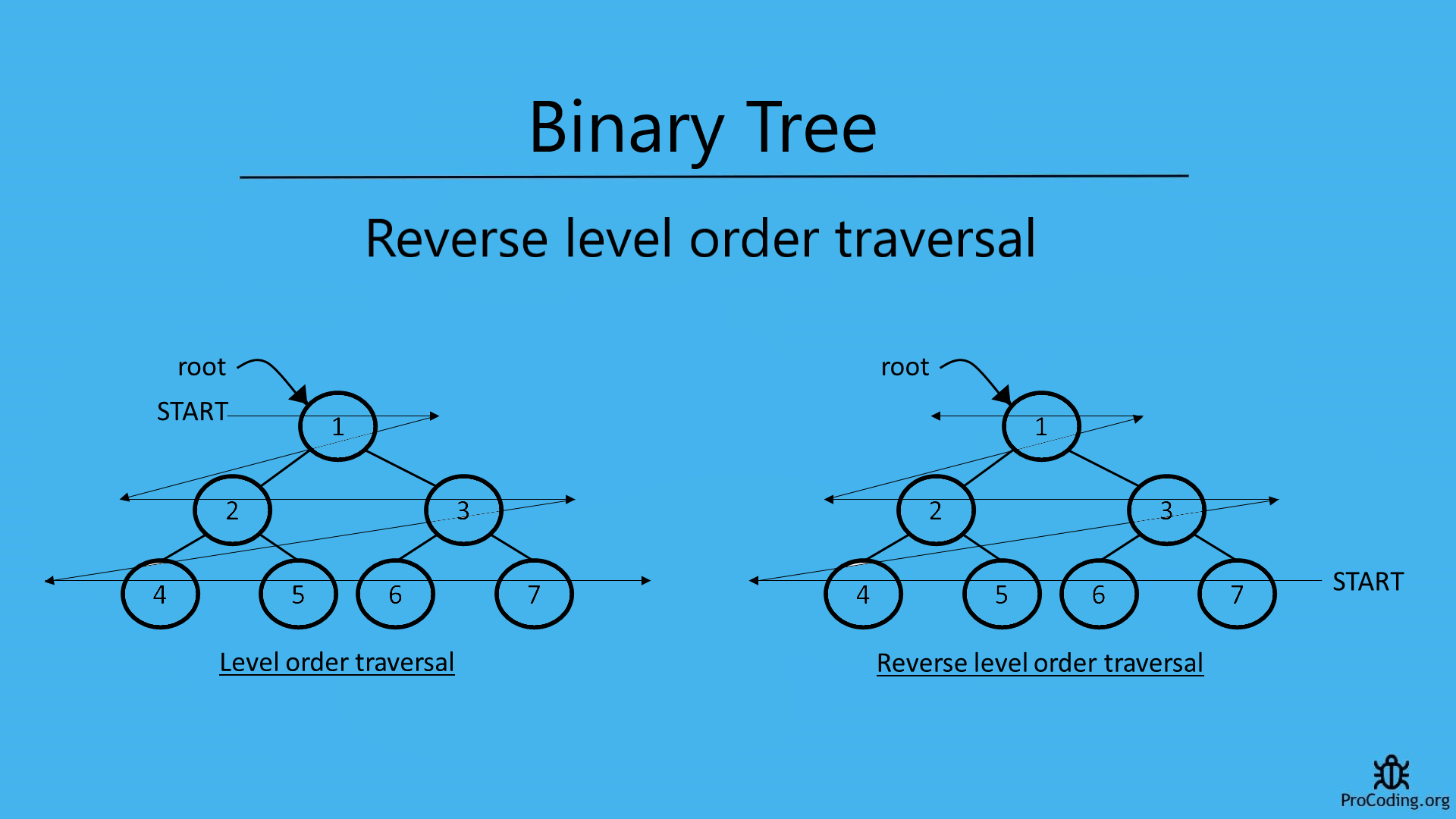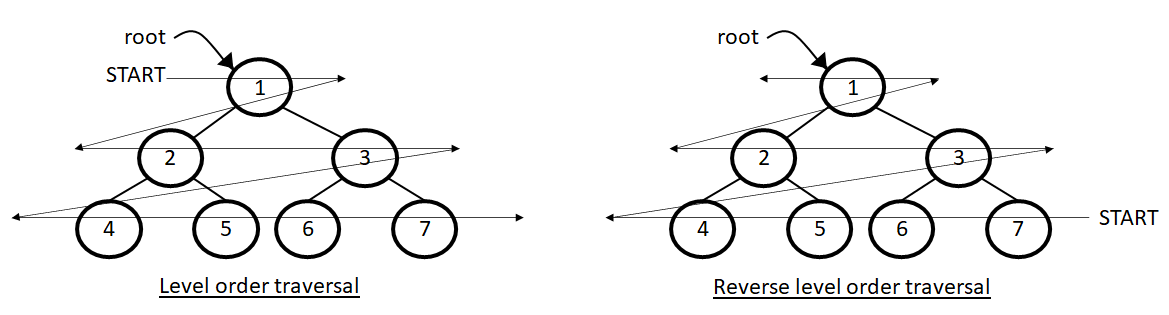# Reverse level order traversal

Category: Data Structures
Tags: #datastructures#binary-tree

Program to print reverse level order traversal of a binary tree i.e., print data in a binary tree from bottom to top and right to left which is just opposite of normal level order traversal of a binary tree.In level order order traversal we traverse each level one by one from top to bottom and left to right. But, in reverse level order traversal we have to traverse from bottom to top and right to left.This can be achieved easily by using same approach as we did for normal level order traversal but here we have to use an extra data structure i.e, stack to store the level order traversal and then print it in reverse order.

Here, we are using stack because in stack we can easily reverse the order of data just by pushing and popping the data.

Python
``````import queue

class Node:
def __init__(self, data):
self.left = None
self.data = data
self.right = None

def level_order_reverse(root):
if root:
q = queue.Queue()
stack = []
q.put(root)

while not q.empty():
node = q.get()
stack.append(node)
if node.left:
q.put(node.left)
if node.right:
q.put(node.right)

while stack:
print(stack.pop().data)

root = Node(1)
root.left = Node(2)
root.right = Node(3)
root.left.left = Node(4)
root.left.right = Node(5)
root.right.left = Node(6)
root.right.right = Node(7)
level_order_reverse(root)
``````
JavaScript
``````class Node {
constructor(data) {
this.left = null;
this.data = data;
this.right = null;
}
}

function levelOrderReverse(root) {
if (root) {
const q = [];
const stack = [];
q.push(root);

while (q.length > 0) {
const node = q.shift();
stack.push(node);
if (node.left) {
q.push(node.left);
}
if (node.right) {
q.push(node.right);
}
}

while (stack.length > 0) {
console.log(stack.pop().data);
}
}
}

const root = new Node(1);
root.left = new Node(2);
root.right = new Node(3);
root.left.left = new Node(4);
root.left.right = new Node(5);
root.right.left = new Node(6);
root.right.right = new Node(7);
levelOrderReverse(root);
``````

Output

``````7
6
5
4
3
2
1
``````